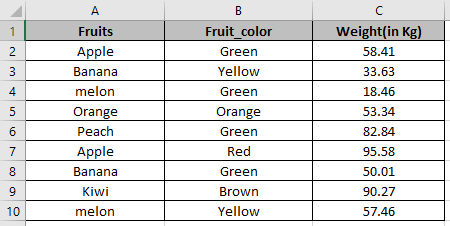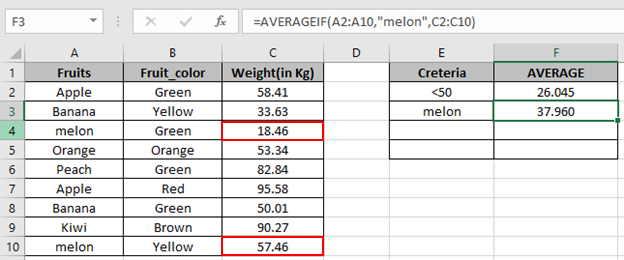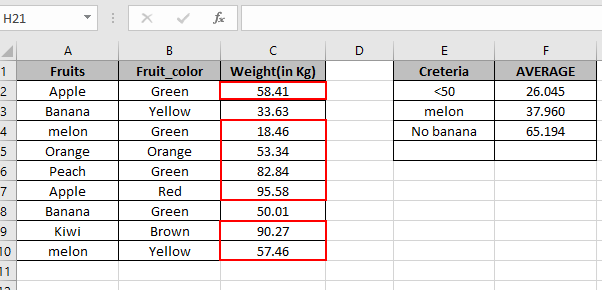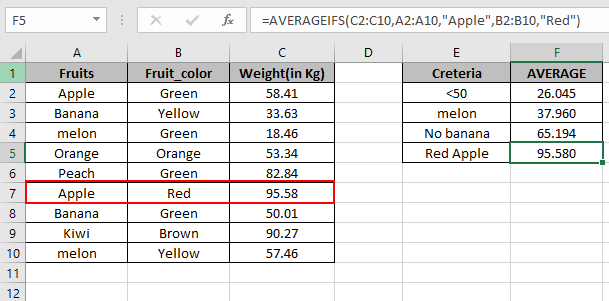# How to use the AVERAGEIF Function in Excel

In this article, we will learn how to get the Average of a list having constraints.
AVERAGEIF function commutes the average of the array that meet the supplied statement.
Syntax:

=AVERAGEIF (range, criteria, [average_range])

range: where criteria is applied.
criteria: condition to be applied
[average range]: [optional]range where the average need to be calculated, if it is different from the first range provided as argument

This is an interesting function to find the average considering the conditions. Let’s see an example below.
Here we have a list of fruits with color and their weight.First we will find out the average of overall weight of the fruits having condition Use the formula

=AVERAGEIF(C2:C10,"<50")

C2:C10 : range where condition (cell_value<50) satisfies.
C2:C50 : the average range as no other range is provided.We got the Average of the cells less than 50.
Now we will compute the Average of the weight having fruit melon.
Use the formula

=AVERAGEIF(A2:A10,"melon",C2:C10)We got the Average of the cells in colored box.
Now we will compute the Average of the weight not having fruit Banana

Use the formula

=AVERAGEIF(A2:A10,"<>Banana",C2:C10)Now we will compute the Average of the weight not having fruit Banana

Use the formula

=AVERAGEIF(A2:A10,"<>Banana",C2:C10)We got the Average of the cells in Red colored box.

Now we will compute the Average of the weight having multiple conditions. We need to use AVERAGEIFS function.
Conditions

1. Fruit Apple
2. Color Red

Use the formula

=AVERAGEIFS(C2:C10, A2:A10,"Apple", B2:B10,"Red")As you can see your Average is here.

Hope you learnt how to use AVERAGEIF function from the above article. Explore more articles here on mathematical formulation. Please feel free to state your queries in the comment box below.

Related Articles:

How to Calculate Mean in Excel

Ignore zero in the Average of numbers

Calculate Weighted Average

Average Difference between lists

Popular Articles:

How to use the VLOOKUP Function in Excel

How to use the COUNTIF function in Excel 2016

How to Use SUMIF Function in Exce

Terms and Conditions of use

The applications/code on this site are distributed as is and without warranties or liability. In no event shall the owner of the copyrights, or the authors of the applications/code be liable for any loss of profit, any problems or any damage resulting from the use or evaluation of the applications/code.